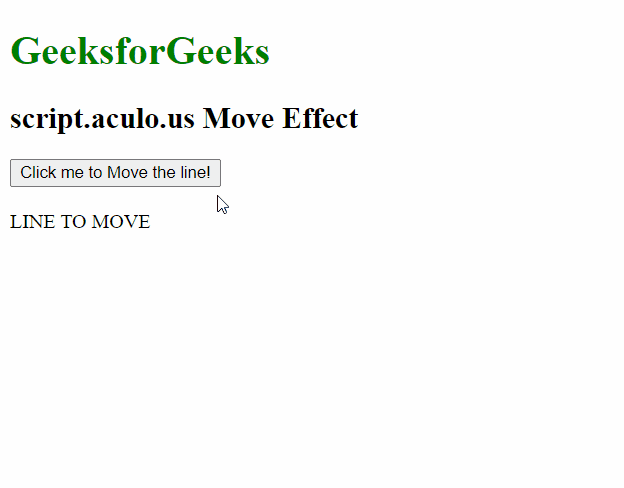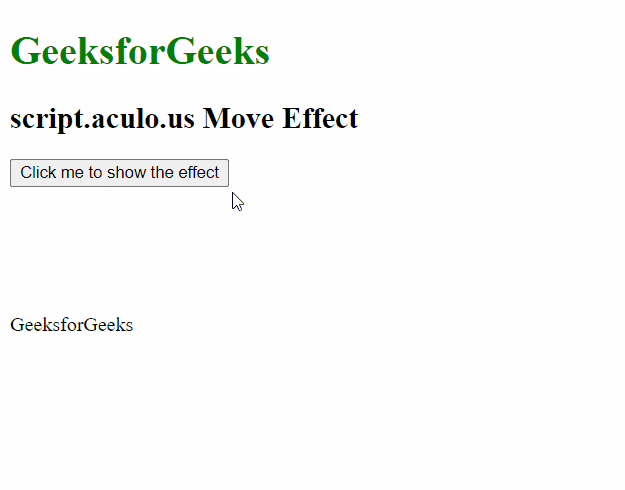# script.aculo.us Move Effect

• Last Updated : 20 Nov, 2020

The script.aculo.us library is a cross-browser library that aims to improving the user interface of a website. In this article, we will demonstrate the Move effect. This effect is used for making the element smoothly move to the given parameters. We can adjust the duration of the effect as well.

Syntax:

`Effect.Move('object', [options] )`

Parameters: This effect has three parameters in the options object described below:

• x: It is an integer that represents the new left value, either absolutely or relatively depending on the mode.
• y: It is an integer that represents the new top value, either absolutely or relatively depending on the mode.
• mode: It is a string that specifies if the element is moved absolutely or relative to its own position.

To demonstrate the use of this function, we have written a small piece of code. In which we have written a small JavaScript function named ShowEffect method which uses the Move method of this library. The examples below demonstrate the method.

Example 1:

## HTML

 ```<``html``>`` ` `<``head``>``    ``<``script` `type``=``"text/javascript"` `        ``src``=``"prototype.js"``>``    ```` ` `    ``<``script` `type``=``"text/javascript"` `        ``src``=``"scriptaculous.js"``>``    ```` ` `    ``<``script` `type``=``"text/javascript"``>``        ``function ShowEffect(element) {`` ` `            ``// Using the Move effect to``            ``// move the element to the``            ``// given absolute position``            ``new Effect.Move(element, {``                ``x: 0,``                ``y: 0,``                ``mode: 'absolute'``            ``});``        ``}``    `````` ` `<``body``>``    ``<``h1` `style``=``"color: green;"``>``        ``GeeksforGeeks``    ```` ` `    ``<``h2``>script.aculo.us Move Effect``     ` `    ``<``button` `onclick``=``"ShowEffect('hideshow')"``>``        ``Click me to Move the line!``    ````     ` `    ``<``br``><``br``>``    ``<``div` `id``=``"hideshow"` `        ``style``=``"position: absolute;"``>``        ``LINE TO MOVE``    `````` ` ``

Output:Example 2:

## HTML

 ```<``html``>`` ` `<``head``>``    ``<``script` `type``=``"text/javascript"` `        ``src``=``"prototype.js"``>``    ```` ` `    ``<``script` `type``=``"text/javascript"` `        ``src``=``"scriptaculous.js"``>``    ```` ` `    ``<``script` `type``=``"text/javascript"``>``        ``function ShowEffect(element) {`` ` `            ``// Using the Move effect to``            ``// move the element to the``            ``// given relative position``            ``new Effect.Move(element, {``                ``x: 50,``                ``y: -50,``                ``mode: 'relative'``            ``});``        ``}``    `````` ` `<``body``>``    ``<``h1` `style``=``"color: green;"``>``        ``GeeksforGeeks``    ```` ` `    ``<``h2``>script.aculo.us Move Effect``     ` `    ``<``button` `onclick``=``"ShowEffect('geeks_1')"``>``        ``Click me to show the effect``    ````     ` `    ``<``br``><``br``>``    ``<``div` `id``=``"geeks_1"` `style``=``        ``"position: absolute; top: 250px"``>``        ``GeeksforGeeks``    `````` ` ``

Output:My Personal Notes arrow_drop_up